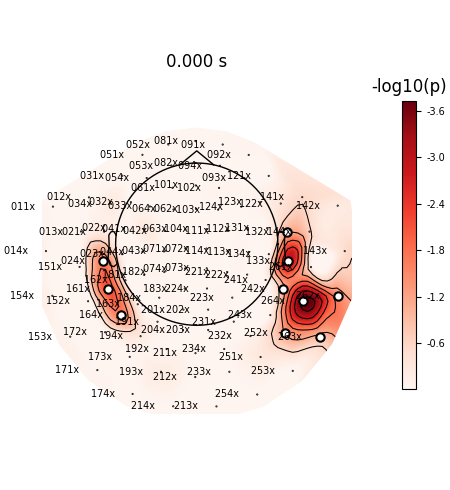# Permutation T-test on sensor data#

One tests if the signal significantly deviates from 0 during a fixed time window of interest. Here computation is performed on MNE sample dataset between 40 and 60 ms.

```# Authors: Alexandre Gramfort <alexandre.gramfort@inria.fr>
#
```
```import numpy as np

import mne
from mne import io
from mne.stats import permutation_t_test
from mne.datasets import sample

print(__doc__)
```

Set parameters

```data_path = sample.data_path()
meg_path = data_path / 'MEG' / 'sample'
raw_fname = meg_path / 'sample_audvis_filt-0-40_raw.fif'
event_fname = meg_path / 'sample_audvis_filt-0-40_raw-eve.fif'
event_id = 1
tmin = -0.2
tmax = 0.5

#   Setup for reading the raw data

picks = mne.pick_types(raw.info, meg='grad', eeg=False, stim=False, eog=True,
epochs = mne.Epochs(raw, events, event_id, tmin, tmax, picks=picks,
data = epochs.get_data()
times = epochs.times

temporal_mask = np.logical_and(0.04 <= times, times <= 0.06)
data = np.mean(data[:, :, temporal_mask], axis=2)

n_permutations = 50000
T0, p_values, H0 = permutation_t_test(data, n_permutations, n_jobs=None)

significant_sensors = picks[p_values <= 0.05]
significant_sensors_names = [raw.ch_names[k] for k in significant_sensors]

print("Number of significant sensors : %d" % len(significant_sensors))
print("Sensors names : %s" % significant_sensors_names)
```
```Opening raw data file /home/circleci/mne_data/MNE-sample-data/MEG/sample/sample_audvis_filt-0-40_raw.fif...
Read a total of 4 projection items:
PCA-v1 (1 x 102)  idle
PCA-v2 (1 x 102)  idle
PCA-v3 (1 x 102)  idle
Average EEG reference (1 x 60)  idle
Range : 6450 ... 48149 =     42.956 ...   320.665 secs
72 matching events found
Setting baseline interval to [-0.19979521315838786, 0.0] sec
Applying baseline correction (mode: mean)
4 projection items activated
Rejecting  epoch based on EOG : ['EOG 061']
Rejecting  epoch based on EOG : ['EOG 061']
Rejecting  epoch based on EOG : ['EOG 061']
Rejecting  epoch based on EOG : ['EOG 061']
Rejecting  epoch based on EOG : ['EOG 061']
Rejecting  epoch based on EOG : ['EOG 061']
Rejecting  epoch based on EOG : ['EOG 061']
Rejecting  epoch based on EOG : ['EOG 061']
Rejecting  epoch based on EOG : ['EOG 061']
Rejecting  epoch based on EOG : ['EOG 061']
Rejecting  epoch based on EOG : ['EOG 061']
Rejecting  epoch based on EOG : ['EOG 061']
Rejecting  epoch based on EOG : ['EOG 061']
Rejecting  epoch based on EOG : ['EOG 061']
Rejecting  epoch based on EOG : ['EOG 061']
Rejecting  epoch based on EOG : ['EOG 061']
Permuting 49999 times...
[Parallel(n_jobs=1)]: Using backend SequentialBackend with 1 concurrent workers.
[Parallel(n_jobs=1)]: Done   1 out of   1 | elapsed:    3.6s remaining:    0.0s
[Parallel(n_jobs=1)]: Done   1 out of   1 | elapsed:    3.6s finished
Number of significant sensors : 11
Sensors names : ['MEG 0243', 'MEG 1323', 'MEG 1333', 'MEG 1613', 'MEG 1643', 'MEG 2423', 'MEG 2522', 'MEG 2622', 'MEG 2632', 'MEG 2642', 'MEG 2643']
```

View location of significantly active sensors

```evoked = mne.EvokedArray(-np.log10(p_values)[:, np.newaxis],
epochs.info, tmin=0.)

# Extract mask and indices of active sensors in the layout
stats_picks = mne.pick_channels(evoked.ch_names, significant_sensors_names)
mask = p_values[:, np.newaxis] <= 0.05